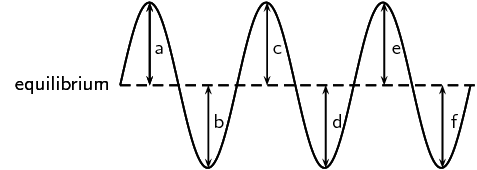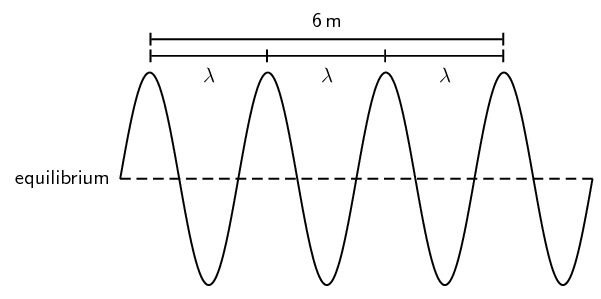Home Practice
For learners and parents For teachers and schools
Textbooks
Full catalogue
Pricing SupportLog in

We think you are located in United States. Is this correct?

# 8.4 Amplitude

## AmplitudeFill in the table below by measuring the distance between the equilibrium and each crest and trough in the wave above. Use your ruler to measure the distances.

 Crest/Trough Measurement (cm) a b c d e f

2. Are the distances between the equilibrium position and each crest equal?

3. Are the distances between the equilibrium position and each trough equal?

4. Is the distance between the equilibrium position and crest equal to the distance between equilibrium and trough?

As we have seen in the activity on amplitude, the distance between the crest and the equilibrium position is equal to the distance between the trough and the equilibrium position. This distance is known as the amplitude of the wave, and is the characteristic height of the wave, above or below the equilibrium position. Normally the symbol A is used to represent the amplitude of a wave. The SI unit of amplitude is the metre (m).

Amplitude

The amplitude of a wave is the maximum disturbance or displacement of the medium from the equilibrium (rest) position.

Quantity: Amplitude (A)         Unit name: metre         Unit symbol: m

A tsunami is a series of sea waves caused by an underwater earthquake, landslide, or volcanic eruption. When the ocean is deep, tsunamis may be less than $$\text{30}$$ $$\text{cm}$$ high on the ocean's surface and can travel at speeds up to $$\text{700}$$ $$\text{km·hr^{-1}}$$. In shallow water near the coast, it slows down. The top of the wave moves faster than the bottom, causing the sea to rise dramatically, as much as $$\text{30}$$ $$\text{m}$$. The wavelength can be as long as $$\text{100}$$ $$\text{km}$$ and the period as long as a hour.

In 2004, the Indian Ocean tsunami was caused by an earthquake that is thought to have had the energy of $$\text{23 000}$$ atomic bombs. Within hours of the earthquake, killer waves radiating from away from the earthquake crashed into the coastline of 11 countries, killing $$\text{150 000}$$ people. The final death toll was $$\text{283 000}$$.

## Worked example 1: Amplitude of sea waves

If the crest of a wave measures $$\text{2}$$ $$\text{m}$$ above the still water mark in the harbour, what is the amplitude of the wave?

### Analyse the information provided

We have been told that the harbour has a still water mark. This is a line created when there are no disturbances in the water, which means that it is the equilibrium position of the water.

### Determine the amplitude

The definition of the amplitude is the height of a crest above the equilibrium position. The still water mark is the height of the water at equilibrium and the crest is $$\text{2}$$ $$\text{m}$$ above this, so the amplitude is $$\text{2}$$ $$\text{m}$$.

## Wavelength

Fill in the table below by measuring the distance between crests and troughs in the wave above.

 Distance(cm) a b c d

2. Are the distances between crests equal?

3. Are the distances between troughs equal?

4. Is the distance between crests equal to the distance between troughs?

As we have seen in the activity on wavelength, the distance between two adjacent crests is the same no matter which two adjacent crests you choose. There is a fixed distance between the crests. Similarly, we have seen that there is a fixed distance between the troughs, no matter which two troughs you look at. More importantly, the distance between two adjacent crests is the same as the distance between two adjacent troughs. This distance is called the wavelength of the wave.

The symbol for the wavelength is λ (the Greek letter lambda) and wavelength is measured in metres (m).

## Worked example 2: Wavelength

The total distance between 4 consecutive crests of a transverse wave is $$\text{6}$$ $$\text{m}$$. What is the wavelength of the wave?

### Draw a rough sketch of the situation### Determine how to approach the problem

From the sketch we see that 4 consecutive crests is equivalent to 3 wavelengths.

### Solve the problem

Therefore, the wavelength of the wave is:

\begin{align*} 3\lambda & = \text{6}\text{ m} \\ \lambda & = \frac{\text{6}\text{ m}}{3} \\ & = \text{2}\text{ m} \end{align*}

The wavelength is $$\text{2}$$ $$\text{m}$$.